Your browser (Unknown 0) is no longer supported. Some parts of the website may not work correctly. Please update your browser.

#### HilbertMaze

Find the shortest path between two fields in a Hilbert maze.

A halfling is searching for treasure hidden in a maze in the dwarfs' mine. He has a map of the maze and would like to find the shortest path to the treasure.

The maze has a specific shape. It is placed on a square grid with M2 cells, where M = 2N+1+1 for some given size N. Each cell has coordinates (x, y), where 0 ≤ x, y < M, and can either be empty or contain a rock.

The mazes of sizes N = 1 and N = 2 are presented in the pictures below: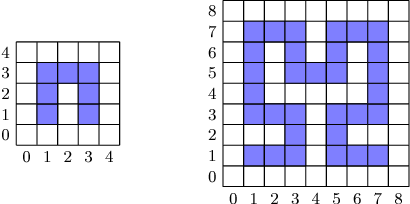A maze of size N is constructed recursively from the layout of the maze of size N−1 (like the Hilbert curve). It contains four mazes of size N−1, each maze in one quarter. The maze in the bottom-left quarter is rotated by 90 degrees clockwise and the maze in the bottom-right quarter is rotated by 90 degrees counter-clockwise. The mazes in the top quarters are not rotated. There are three additional rocks (squares marked in green in the picture below) in the areas where the mazes intersect. The construction of the maze of size N = 3 is shown below: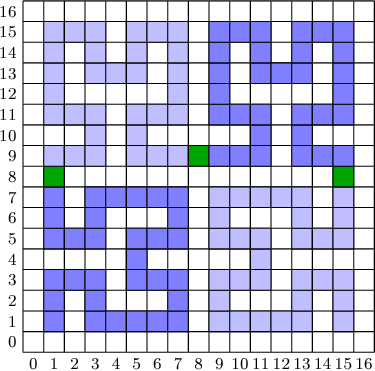The halfling would like to reach the treasure in the smallest number of steps possible. At each step, he can move to any one of the four adjacent cells (north, south, west, east) that does not contain a rock and is not outside of the grid.

For example, given N = 1, the halfling needs 8 steps to move from cell (2, 1) to cell (3, 4):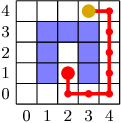Write a function:

class Solution { public int solution(int N, int A, int B, int C, int D); }

that, given the size of the maze N, coordinates of the halfling (A, B) and coordinates of the treasure (C, D), returns the minimum number of steps required for the halfling to reach the treasure.

## Examples

Given N = 1, A = 2, B = 1, C = 3 and D = 4 the function should return 8, as shown above.

Given N = 2, A = 2, B = 5, C = 6 and D = 6 the function should return 7: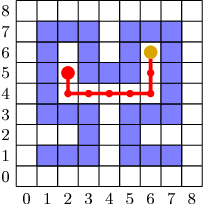Given N = 3, A = 6, B = 6, C = 10 and D = 13 the function should return 39: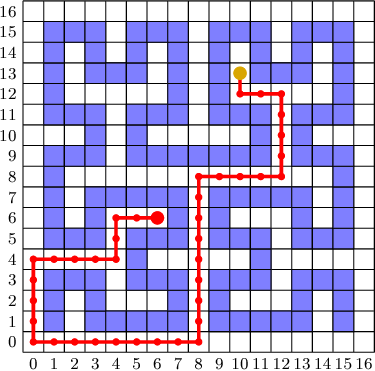Write an efficient algorithm for the following assumptions:

• N is an integer within the range [1..25];
• A, B, C, D are integers within the range [0..2N+1];
• cells (A, B) and (C, D) do not contain rocks;
• the result will be an integer smaller than 2,147,483,647.